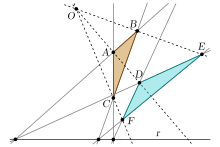Main subject: Math
related subject: Math
Duration: 30 minutes.
Age groups: Age 10
Submitted by: Dagmar Þóra Sævarsdóttir og Lísbet Patrisía Gísladóttir. Iceland.

### Learning objectives:

Learning objectives are: Lesson 1 in Geometry, area of a rectangle and perimeter. The students review/learn how to find an area of a rectangle, review/learn what is called a square, length and width and how to find the perimeter of an area. They review/learn to count squares and multiply length by the width to find how big an area is.

### Implemented digital tools:

Device with internet connection. One for the teacher and one for each student or group. Digital tool is an webside, http://rasmus.is/

Problem solving

### Elaboration of the competences:

To find out if the students understand the material that has been teached and review the material.

### Preparation:

The students gets their digital devices and go to the webside, http://rasmus.is/ and choose the language you want them to work with. Then they press start and choose grade primary. The teacher can print out ,,Your Checklist” for each student to write down the results.

### introduction

The students choose Geometry and open Lesson 1.

### Implementation:

When the students have open Lesson 1 they read the introduction about: Area of rectangle, Calculating area and Calculating the perimeter. When they are finished the students choose Quiz 1 and send their answers to get their results.

### Conclusion:

The students review their results, write it down and show it to the teacher. If there are some incorrect answers the students try to solve them with their teacher.